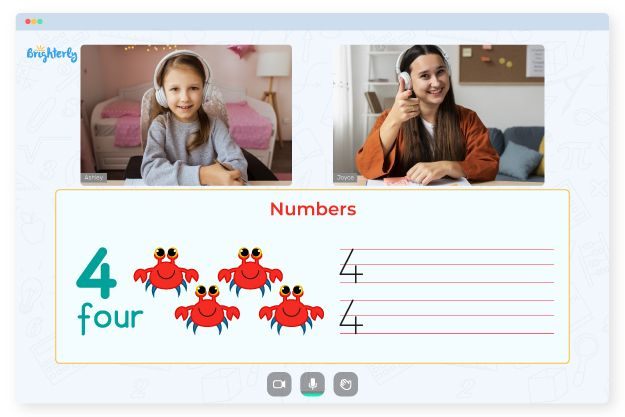Solving quadratic inequalities worksheets help students understand that an inequality sign replaces the equal sign in a second-degree quadratic equation. The idea of quadratic inequalities is fundamental to the study of mathematics. For example, quadratic inequalities help compare numbers and find the range of values that fulfill the condition of a particular variable.

Math for Kids

Is Your Child Struggling With Math?
1:1 Online Math TutoringRegular practice worksheet quadratic inequalities boosts children’s problem-solving abilities. In addition, using these resources as a standard practice tool will heighten students’ self-confidence and grasp of mathematics.

Parents can also use these worksheets to ascertain their kids’ abilities so they can get an insight into how to support a child’s development. Furthermore, tutors and parents can teach students how to learn independently with the paper worksheet.Trouble with Numbers?• Does your child have trouble with numbers?
• Start studying with an online tutor.

Is your child having difficulties understanding numbers? An online tutor could be the answer.